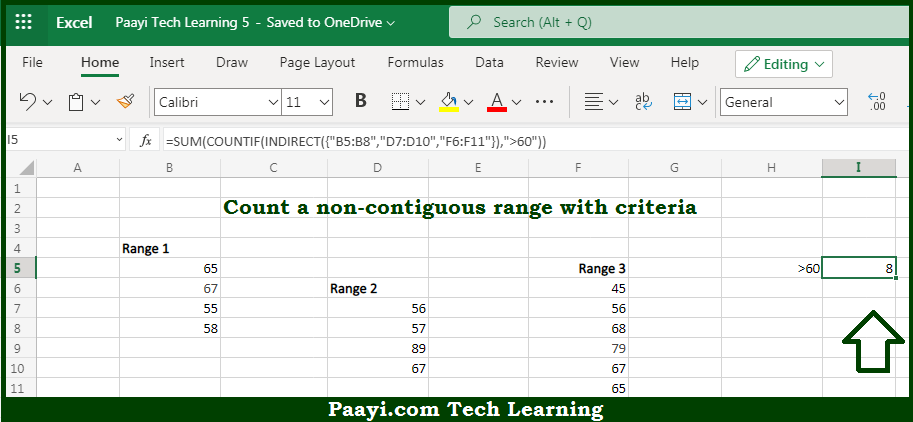# Learn How to COUNTIF With Non Contiguous Range in Microsoft Excel

Written by | 0 Comments | 795 Views

In this article, you will learn how to COUNT various things in Microsoft Excel using a single or combination of functions and its purpose. You will also get to know how to COUNTIF With Non-Contiguous Range and see the generic formula.

COUNTIF With Non-Contiguous Range in Microsoft Excel

The main purpose of this formula is to count a non-contiguous range with criteria. Here we will learn how to count with a non-contiguous range using COUNTIF with given criteria in Microsoft Excel. That implies, with the help of a formula based on the COUNTIF, INDIRECT, and SUM function you can able to count a non-contiguous range with criteria. So, with the help of this formula, you can able to count with a non-contiguous range using COUNTIF with given criteria.

General Formula to COUNTIF With Non-Contiguous Range

=SUM(COUNTIF(INDIRECT({"rng1","rng2","rng3"}),criteria))

The Explanation for the COUNTIF With Non-Contiguous RangeSo we know that with the help of the given formula above you can able to count a non-contiguous range with criteria. Here we will learn how to count with a non-contiguous range using COUNTIF with given criteria in Microsoft Excel. As we know that the COUNTIF function is used to count the number of cells in a range that meets the given criteria. In case you want to use the COUNTIF function with multiple ranges separated by commas, you'll get an error. The solution here could be to write out the ranges as text in an array constant inside the INDIRECT function. So, with the help of this formula, you can able to count with a non-contiguous range using COUNTIF with given criteria.